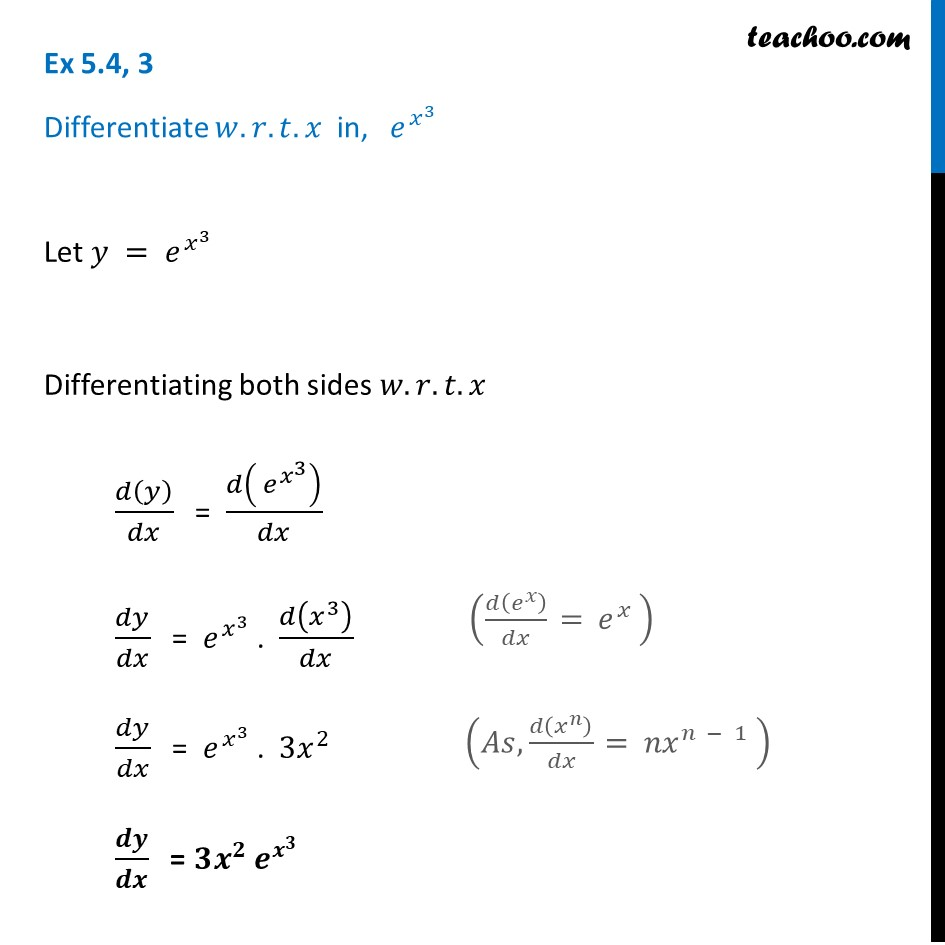Ex 5.4

Chapter 5 Class 12 Continuity and Differentiability
Serial order wiseLearn in your speed, with individual attention - Teachoo Maths 1-on-1 Class

### Transcript

Ex 5.4, 3 Differentiate 𝑤.𝑟.𝑡. 𝑥 in, 〖 𝑒〗^(𝑥^3 ) Let 𝑦 =〖 𝑒〗^(𝑥^3 ) " " Differentiating both sides 𝑤.𝑟.𝑡.𝑥 𝑑(𝑦)/𝑑𝑥 = 𝑑(〖 𝑒〗^(𝑥^3 ) )/𝑑𝑥 𝑑𝑦/𝑑𝑥 = 〖 𝑒〗^(𝑥^3 ) . 𝑑(𝑥^3 )/𝑑𝑥 𝑑𝑦/𝑑𝑥 = 〖 𝑒〗^(𝑥^3 ) . 3𝑥^2 𝒅𝒚/𝒅𝒙 = 𝟑𝒙^𝟐 〖 𝒆〗^(𝒙^𝟑 )